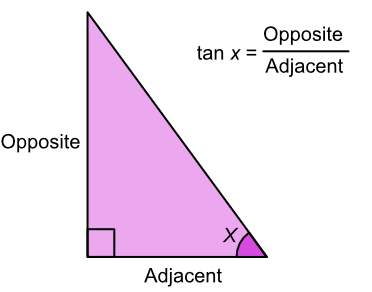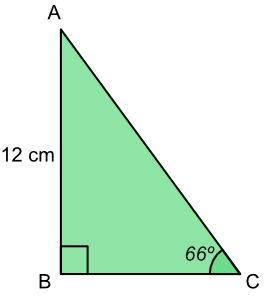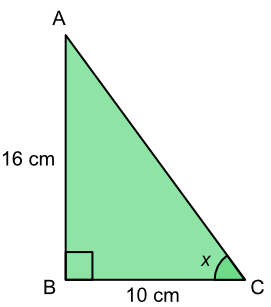Tan

# Tan

GCSE(F), GCSE(H),

The ratio of the opposite side to the adjacent is the tangent, or tan of the angle. The adjacent side is the non-hypotenuse side that touches the angle: the opposite side is opposite the angle.If an angle and one length is known, find the tan of the angle and substitute this value into the formula along with the known length.

If the lengths are known, but not the angle, then the formula gives the tangent of the angle.The value of the angle can be obtained using the inverse operation (tan-1 on a calculator).

## Examples

1. What is the length of BC?tan x = frac(text(opposite))(text(adjacent))

tan 66 = frac(12)(text(BC))

(BC) x 2.246 = 12

BC = 12 ÷ 2.246

AB = 5.342

2. What is the value of the angle x?tan x = frac(text(opposite))(text(adjacent))
tan x = frac(16)(10)